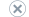# MDX Query and Slicer Axes - Using Axes in a Simple Example

Applies to:SQL Server Analysis ServicesAzure Analysis ServicesPower BI Premium

The simple example presented in this topic illustrates the basics of specifying and using query and slicer axes.

## The Cube

A cube, named TestCube, has two simple dimensions named Route and Time. Each dimension has only one user hierarchy, named Route and Time respectively. Because the measures of the cube are part of the Measures dimension, this cube has three dimensions in all.

## The Query

The query is to provide a matrix in which the Packages measure can be compared across routes and times.

In the following MDX query example, the Route and Time hierarchies are the query axes, and the Measures dimension is the slicer axis. The Members function indicates that MDX will use the members of the hierarchy or level to construct a set. The use of the Members function means that you do not have to explicitly state each member of a specific hierarchy or level in an MDX query.

``````SELECT
{ Route.nonground.Members } ON COLUMNS,
{ Time.[1st half].Members } ON ROWS
FROM TestCube
WHERE ( [Measures].[Packages] )
``````

## The Results

The result is a grid that identifies the value of the Packages measure at each intersection of the COLUMNS and ROWS axis dimensions. The following table shows how this grid would look.

air sea
1st quarter 60 50
2nd quarter 45 45